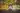# 突破防采集，再难也要上(有人说楼主是骗纸，网页根本打不开呀!) (擦，此站和类似的其他网站需要翻墙)```var _html = []; \$('#listtable tbody tr' ).each(function(i, n){ var _line = []; var _self = \$(this); //var tds = _self.find('td') _line.push(_self.find('td:eq(1)').text()); _line.push(_self.find('td:eq(2)').text()); _html.push(_line.join(':')); }) console.log(_html.join('\n'));```

```.UBXR{display:none} .rcBy{display:inline} .wikF{display:none} .wECt{display:inline} 4545188218.18140.208.66136: 9000 .UbG7{display:none} .odO4{display:inline} .uK-0{display:none} .cvKi{display:inline} .CiSC{display:none} .azWI{display:inline} 109156156.585877939595130130130175184184184196196211.6668232929187187246.8888135135135136174240: 8080 .j05u{display:none} .A5AR{display:inline} .X9qd{display:none} .qnVe{display:inline} .ylFA{display:none} .V_RW{display:inline} .eqQH{display:none} .Wfut{display:inline} 505093121213214214.110147147.23232632515161188196203203.133154195: 8080``````var _html = []; \$('#listtable tbody tr' ).each(function(i, n){ var _line = []; var _self = \$(this); //var tds = _self.find('td') var td = _self.find('td:eq(1)'); td.find('style,span:hidden,div:hidden').remove(); //注意这里 _line.push(td .text()); _line.push(_self.find('td:eq(2)').text()); _html.push(_line.join(':')); }) console.log(_html.join('\n'));``````static class CssStreamTokenizer extends StreamTokenizer { public CssStreamTokenizer(Reader r) { super(r); this.resetSyntax(); this.wordChars(0, 255); this.ordinaryChar('.'); this.ordinaryChar('#'); this.ordinaryChar('{'); this.ordinaryChar('}'); } static final int CSS_EOF = -0x01; static final int CSS_BLOCK = -0x02; static final int CSS_CLASS = -0x04; static final int CSS_ID = -0x08; static final int CSS_UNKNOWN = -0x10; boolean isOutClass = true; boolean isOutId = true; boolean isOutBlock = true; static final Pattern P_CLASS = Pattern.compile("^[a-zA-Z][\\w\\-]*"); static final Pattern P_ID = Pattern.compile("^[a-zA-Z][\\w\\-\\:]*"); public int nextCSS() { int token; try{ switch(token = this.nextToken()){ case StreamTokenizer.TT_EOF: return CSS_EOF; case '{': //进入标记字段 isOutBlock = false; return nextCSS(); case '}': isOutBlock = true; isOutClass = true; isOutId = true; return nextCSS(); case '.': isOutClass = false; return nextCSS(); case '#': isOutId = false; return nextCSS(); case StreamTokenizer.TT_WORD: if(!isOutId && isOutBlock && P_ID.matcher(sval).find()) return CSS_ID; else if(!isOutClass && isOutBlock && P_CLASS.matcher(sval).find()) return CSS_CLASS; else if (!isOutBlock) return CSS_BLOCK; else return CSS_UNKNOWN; default: return CSS_UNKNOWN; } //end of case }catch(IOException e){ System.out.println("Error:"+e.getMessage()); } return CSS_UNKNOWN; } }```

CSSStreamTokenizer 的主要功能就像他的名字一样，把 CSS 一串字符串，按照语法切分成语法的组成部分，可以得到 CLASS 、 ID和具体内容。利用 CSSStreamTokenizer 可以轻易的分析一串 CSS 中，哪个 class 是 hidden的，接着看下面的 Parser,

```static class CssParser { static final Pattern P_HIDDEN_CSS = Pattern.compile("display\\s*[\\:]\\s*none"); private String html; CssParser(String html) { this.html = html; } public String[] getHiddenClasses() { if (html == null || html.length() == 0) return null; CssStreamTokenizer tokenizer = new CssStreamTokenizer(new StringReader(html)); int token = 0; boolean inCSS = false; Stack stack = new Stack(); while ((token = tokenizer.nextCSS()) != CssStreamTokenizer.CSS_EOF) { String sval = tokenizer.sval; if (token == CssStreamTokenizer.CSS_CLASS) { System.out.println(">>CLASS"); inCSS = true; stack.push(sval); // push first } else if (token == CssStreamTokenizer.CSS_ID) { System.out.println(">>ID"); } else if (token == CssStreamTokenizer.CSS_BLOCK) { System.out.println(">>BLOCK"); if (inCSS && !P_HIDDEN_CSS.matcher(sval).find()) { stack.pop(); // pop it, not hidden class } inCSS = false; } else { System.out.println(">>UNKNOWN"); inCSS = false; } System.out.println(tokenizer.sval); } return stack.toArray(new String[]{}); } }```

Bill 2014-01-05

(本站文章均为原创，侵权必究)